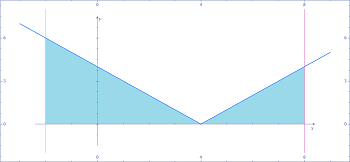# Sketch the region whose area is represented by the definite integral. Integral from -2 to 8 of...

## Question:

Sketch the region whose area is represented by the definite integral.

{eq}\int_{-2}^{8} \left | x-4 \right | \, \mathrm{d}x {/eq}

Use a geometric formula to evaluate the integral.

## Area:

Recall that the area under a positive curve {eq}y=f(x),\, a\leq x\leq b {/eq} is given by the definite integral {eq}\int_a^b f(x)\, dx {/eq}. This was our original motivation for defining the integral and it allows us to evaluate the area under curves without standard geometric formulas.

The region whose area is given by the integral {eq}\int_{-2}^8 \left| x-4\right| \, dx {/eq} is shown belowWe also recall that the area of a...

Become a Study.com member to unlock this answer! Create your account